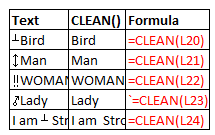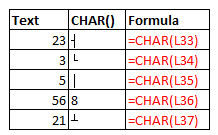Excel Text functions: asc, clean, char, code, dollar

Excel Function ASC

The ASC() function is used with Double byte character set (DBCS).

This function changes the double byte char into single byte characters.

There are a number of Unicode characters that uses 2 bytes per character instead of single byte as in ASCII.

This function is most helpful with the above said encodings.

 Text ASC Formula Bird Bird =ASC(L4) a a =ASC(L5) boy boy =ASC(L6) woman woman =ASC(L7) lady lady =ASC(L8)

As seen from the above screenshot, there is no difference between actual text and its ASC counterpart.

This is because, English characters are already single byte or half width characters.

Excel Function CLEAN

The CLEAN() function is used to remove any non printable characters from a given text.

The non printable characters includes line breaks, tab space and so on.

This function is often used with print operations for better look.

The ASCII code corresponding to these symbols range from 0 to 31.Excel Function CHAR

The CHAR() function is used to convert the ASCII value specified to the corresponding character.

This function is most commonly used with third party files, wherein data are encoded in ASCII.

This function takes single parameter; a number or ASCII code.Text CHAR() Formula 90 Z =CHAR(L33) 46 . =CHAR(L34) 125 } =CHAR(L35) 56 8 =CHAR(L36) 145 � =CHAR(L37)

Excel Function CODE()

The CODE() function is used to convert the first charater of a string into its ASCII code.

This function can be supplied with either a single charater or a text.

In either case, only the first character is considered by this function.

 Text CODE() Formula Zbird 90 =CODE(L45) Man 77 =CODE(L46) H 72 =CODE(L47) 8 56 =CODE(L48) I am Strong!! 73 =CODE(L49)

Excel Function DOLLAR()

The DOLLAR() function is used to convert a given numerical value to the currency format with a specified

number of decimal places.

The default currency format of the machine is used by this function.

This function takes two parameters: number, decimal places.

 Number DOLLAR() Formula 56 CHF 56.00 =DOLLAR(L57,2) 78.25 CHF 78.3 =DOLLAR(L58,1) 25 CHF 25.000 =DOLLAR(L59,3) 34 CHF 34 =DOLLAR(L60,0) 100 CHF 100.00 =DOLLAR(L61)